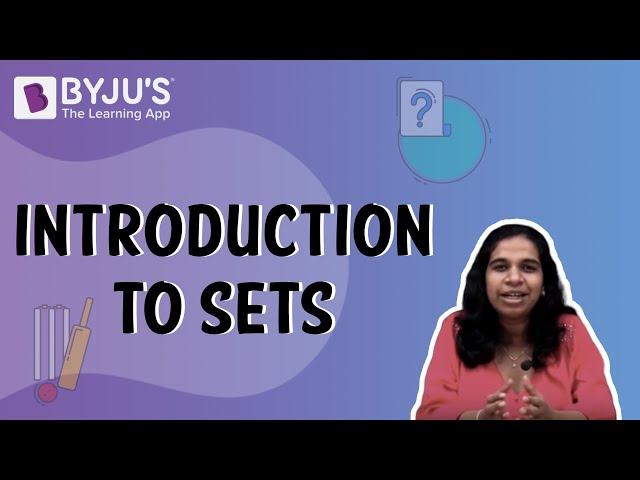# Types Of Sets

Set is defined as a well-defined collection of objects. These objects are referred to as elements of the set. Different types of sets are classified according to the number of elements they have.  Basically, sets are the collection of distinct elements of the same type. For example, a basket of apples, a tea set, a set of real numbers, natural numbers, etc. Let us learn the types of sets here in this article.## Types of Sets in Maths

The different types of sets are as follows:

### Empty Set

The set is empty! This means that there are no elements in the set. This set is represented by ϕ or {}. An empty set is hence defined as:

Definition: If a set doesn’t have any elements, it is known as an empty set or null set or void set. For e.g. consider the set,

P = {x : x is a leap year between 1904 and 1908}

Between 1904 and 1908, there is no leap year. So, P = ϕ.

Similarly, the set,

Q = {y : y is a whole number which is not a natural number,y ≠ 0}

0 is the only whole number that is not a natural number. If y ≠ 0, then there is no other value possible for y. Hence, Q = ϕ.

### Singleton Set

If a set contains only one element, then it is called a singleton set. For e.g.

A = {x : x is an even prime number}

### Finite Set

In this set, the number of elements is finite. All the empty sets also fall into the category of finite sets.

Definition: If a set contains no element or a definite number of elements, it is called a finite set.

If the set is non-empty, it is called a non-empty finite set. Some examples of finite sets are:
A = {x : x is a month in a year}; Set A will have 12 elements

B={y: y is the zero of a polynomial x4 -6x2 + x+ 2}; Set B will have 4 zeroes

### Infinite Set

Just contrary to the finite set, it will have infinite elements. If a given set is not finite, then it will be an infinite set.

For e.g.
A = {x : x is a natural number}; There are infinite natural numbers. Hence, A is an infinite set.

B = {y: y is the ordinate of a point on a given line}; There are infinite points on a line. So, B is an infinite set.

### Power Set

An understanding of what subsets are is required before going ahead with Power-set.

Definition: The power set of a set A is the set which consists of all the subsets of the set A. It is denoted by P(A).

For a set A which consists of n elements, the total number of subsets that can be formed is 2n . From this, we can say that P(A) will have 2n elements.

Example: If set A = {-9,13,6}, then power set of A will be:

P(A)={ϕ, {-9}, {13}, {6}, {-9,13}, {13,6}, {6,-9}, {-9,13,6}}

### Sub Set

If A={-9,13,6}, then,

Subsets of A= ϕ, {-9}, {13}, {6}, {-9,13}, {13,6}, {6,-9}, {-9,13,6}

Definition: If a set A contains elements which are all the elements of set B as well, then A is known as the subset of B.

### Universal Set

This is the set which is the base for every other set formed. Depending upon the context, the universal set is decided. It may be a finite or infinite set. All the other sets are the subsets of the Universal set. It is represented by U.

For e.g. The set of real numbers is a universal set of integers, rational numbers, and irrational numbers.

## Video Lesson on What are SetsIn the discussion above, we have learned how to classify sets on the basis of their elements. To learn more about sets and other topics, visit our site BYJU’S and find interesting articles on every topic.

## Frequently Asked Questions – FAQs

Q1

### What is an empty set?

If a set doesn’t have elements, it is known as an empty set, null set, or void set.
Q2

### What is Singleton Set?

If a set contains only one element, then it is called a singleton set.
Q3

### What is a Finite Set?

If a set contains no element or a definite number of elements, it is called a finite set.
Q4

### What is a Power Set?

The power set of a set A is the set which consists of all the subsets of set A. It is denoted by P(A).
Q5

### What is Sub Set?

If a set A contains elements which are all the elements of set B as well, then A is known as the subset of B.
Test your knowledge on Types Of Sets